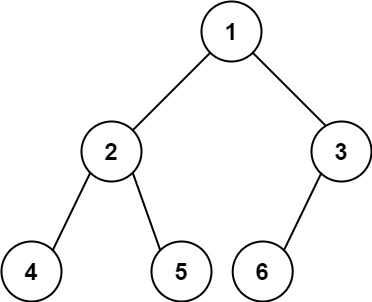222. Count Complete Tree Nodes

Given the `root` of a complete binary tree, return the number of the nodes in the tree.

According to Wikipedia, every level, except possibly the last, is completely filled in a complete binary tree, and all nodes in the last level are as far left as possible. It can have between `1` and `2h` nodes inclusive at the last level `h`.

Example 1:```Input: root = [1,2,3,4,5,6]
Output: 6
```

Example 2:

```Input: root = []
Output: 0
```

Example 3:

```Input: root = 
Output: 1
```

Constraints:

• The number of nodes in the tree is in the range `[0, 5 * 104]`.
• `0 <= Node.val <= 5 * 104`
• The tree is guaranteed to be complete.

Follow up: Traversing the tree to count the number of nodes in the tree is an easy solution but with `O(n)` complexity. Could you find a faster algorithm?

222. Count Complete Tree Nodes
``````struct Solution;
use rustgym_util::*;

trait Count {
fn count(&self) -> i32;
}

fn count(&self) -> i32 {
if let Some(node) = self {
1 + node.borrow().left.count() + node.borrow().right.count()
} else {
0
}
}
}

impl Solution {
fn count_nodes(root: TreeLink) -> i32 {
root.count()
}
}

#[test]
fn test() {
let root = tree!(1, tree!(2, tree!(4), tree!(5)), tree!(3, tree!(6), None));
let res = 6;
assert_eq!(Solution::count_nodes(root), res);
}
``````